# HSPT Math : Arithmetic

## Example Questions

### Example Question #35 : How To Work With Percentages

To get on the ballot for Student Body President, Annie needs the signatures of 5% of the sophomores, 5% of the juniors, and 5% of the seniors.

Annie currently has a petition with the signatures of 22 seniors out of a total of 412, 23 juniors out of a total of 436, and 26 sophomores out of a total of 536. Does Annie have the signatures she needs to get on the ballot?

Possible Answers:

Yes

No; she does not have the signatures of enough sophomores

No; she does not have the signatures of enough seniors

No; she does not have the signatures of enough juniors

Correct answer:

No; she does not have the signatures of enough sophomores

Explanation:

Annie needs the signatures of 5% of the 412 seniors; this is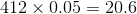,

which means she needs the signatures of at least 21 seniors. She has 22.

She needs the signatures of 5% of the 436 juniors; this is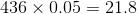,

which means she needs the signatures of at least 22 juniors. She has 23.

She needs the signatures of 5% of the 536 sophomores; this is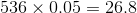.

which means she needs the signatures of at least 27 sophomores. She has 26.

She has enough signatures from seniors and juniors, but not sophomores.

### Example Question #36 : How To Work With Percentages

Four students ran for student body president - Carolyn, Dick, Mike, and Rhonda.

According to the rules, a candidate must win at least 60% of the vote in order to claim an outright victory; otherwise, a runoff must be held between the top two vote-getters. It is known that Carolyn, Dick, and Mike won 87 votes, 56 votes, and 73 votes. It is also known that Rhonda won the election outright. How many votes did Rhonda win at the very least?

Possible Answers: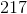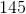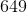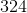Correct answer:Explanation:

At the very least, Rhonda won 60% of the votes cast, so the total number of votes won by her opponents can be at most 40% of the votes cast. Suppose this is the case. If we letbe the number of votes Rhonda won, then we can set up the ratio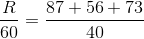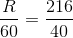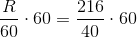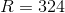At minimum, Rhonda won 324 votes.

### Example Question #37 : How To Work With Percentages

Four students ran for student body president - Benjy, Carla, Hy, and Lisa.

According to the rules, a candidate must win more than 50% of the vote in order to claim an outright victory; otherwise, a runoff must be held between the top two vote-getters.

It is known that Benjy got 56 votes, Carla got 72 votes, and Lisa got 66 votes. It is also known that a runoff must be held between Carla and Lisa. What is the greatest possible percent of the vote that Hy could have gotten?

(Nearest whole percent)

Possible Answers: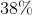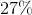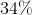Correct answer:Explanation:

Carla and Lisa won the highest and second-highest number of votes, respectively, so Hy must  have won fewer votes than Lisa. He won at most 65 votes. This would have been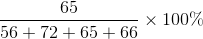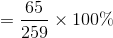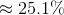The closest answer is 25%.

### Example Question #41 : How To Work With Percentages

To get on the ballot for senior class president, a student must have the signatures of 15% of the senior class. Out of 341 seniors, 52 have signed Clinton's petition. How many more signatures does Clinton need to get on the ballot?

Possible Answers:Clinton has enough signatures to get on the ballot.Correct answer:

Clinton has enough signatures to get on the ballot.

Explanation:

15 % of the 341 members of the senior class is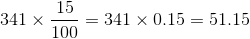.

Since Clinton has 52, he has enough signatures.

### Example Question #11 : How To Solve Arithmetic Word Problems

Bill decides to donate 15% of his $4000 and Ted decides to donate 25% of his$8000. What percentage of their combined fortunes do Bill and Ted donate?

Possible Answers:

23.1%

19.2%

20%

21.7%

22.3%

Correct answer:

21.7%

Explanation:

This is a simple problem, so long as you don't forget that Bill and Ted have different amounts of money, so you can't simply average 15% and 25% to get 20%.

Calculate how much each donates:

4000(.15)= 600

8000(.25)= 2000

Now divide the total donation by their total wealth: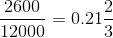Now multiply this answer by 100, and then round to the nearest tenth to get 21.7%.

### Example Question #42 : How To Work With Percentages

If you have a garden in which you planted 120 seeds, what percentage sprouted if you have 90 plants?

Possible Answers: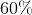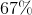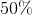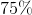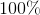Correct answer:Explanation:

To find the percent you must put the part over the whole,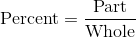Whole is represented by the number of seeds planted and the part represents the number of plants in the garden.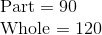Substitute the values into the percent formula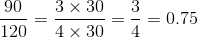Now multiply by 100 to make it a percent which is.

### Example Question #43 : How To Work With Percentages

What percent of 96 is 24?

Possible Answers: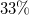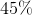Correct answer:Explanation:

Translate the sentence into an equation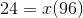Isolateand you get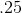.

Remember that the question is asking for a percentage, so convertinto.

### Example Question #1 : How To Add / Subtract / Multiply / Divide Negative Numbers

Which of the following operations always gives a negative result?

Possible Answers:

A negative number divided by a negative number

A negative number subtracted from a negative number

A negative number multipled by a negative number

A negative number taken to the power of a positive integer

A negative number added to a negative number

Correct answer:

A negative number added to a negative number

Explanation:

The sum of two negative numbers is always negative, hence, this is the right choice.

As for the other choices:

The product or quotient of two negative numbers is always positive

A negative number taken to the power of a positive integer can be either negative or positive depending on whether the exponent is even or odd.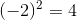, which is positive, and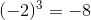, which is negative.

The difference of negative numbers can be either negative, positive, or zero: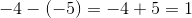, but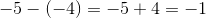### Example Question #1 : Negative Numbers

If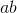is a positive number, and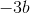is also a positive number, what is a possible value for?

Possible Answers:Correct answer:Explanation:

Because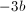is positive,must be negative since the product of two negative numbers is positive.

Because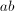is also positive,must also be negative in order to produce a prositive product.

To check you answer, you can try plugging in any negative number for.

### Example Question #3 : How To Add / Subtract / Multiply / Divide Negative Numbers

If x is a negative integer, what else must be a negative integer?

Possible Answers:

x – x

x² – x

x – (–x)

Correct answer:

x – (–x)

Explanation:

By choosing a random negative number, for example: –4, we can input the number into each choice and see if we come out with another negative number.  When we put –4 in for x, we would have –4 – (–(–4)) or –4 – 4, which is –8.  Plugging in the other options gives a positive answer.  You can try other negative numbers, if needed, to confirm this still works.

### All HSPT Math Resources